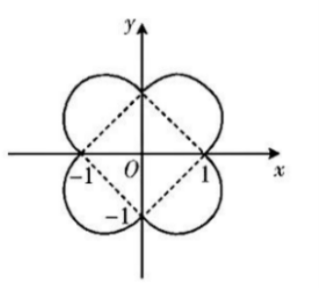【答案】 $\frac{2}{\pi+2} .$

【解析】 当 $x \geqslant 0, y \geqslant 0$ 时, $x^2+y^2=|x|+|y|$ 可化为 $\left(x-\frac{1}{2}\right)^2+\left(y-\frac{1}{2}\right)^2=\frac{1}{2}$;

$$\frac{(\sqrt{2})^2}{4 \times\left(\frac{\sqrt{2}}{2}\right)^2 \pi \times \frac{1}{2}+(\sqrt{2})^2}=\frac{2}{\pi+2} .$$